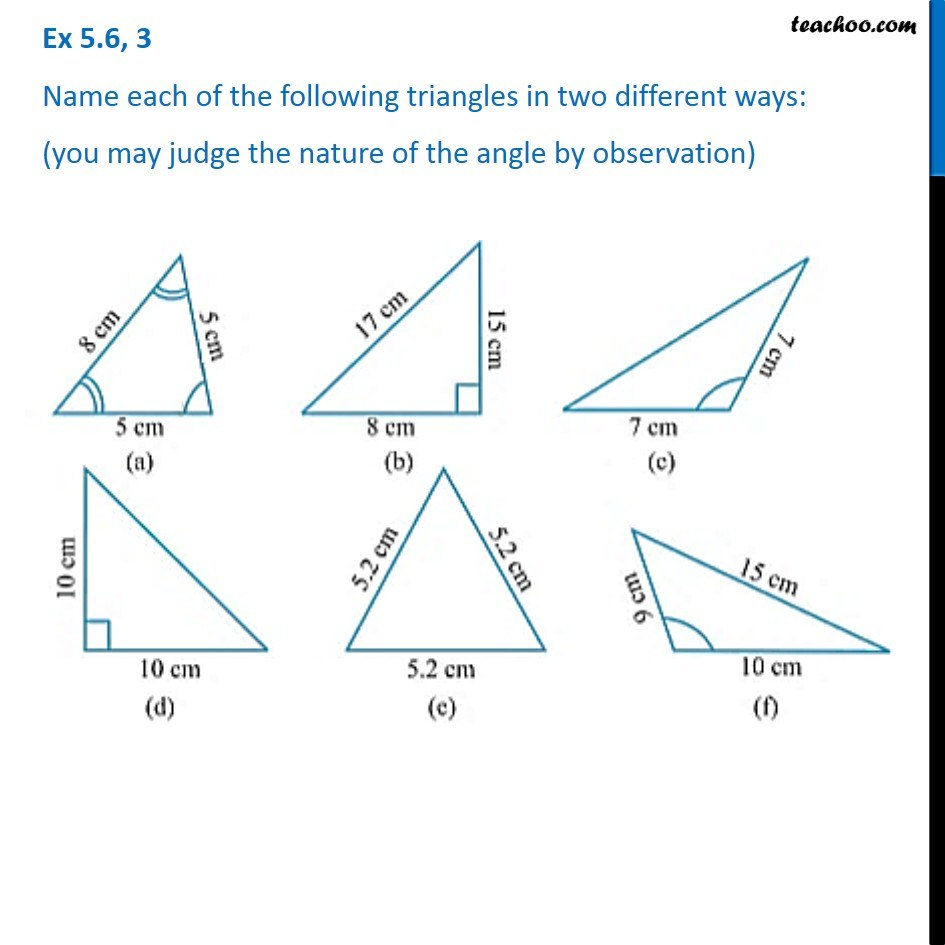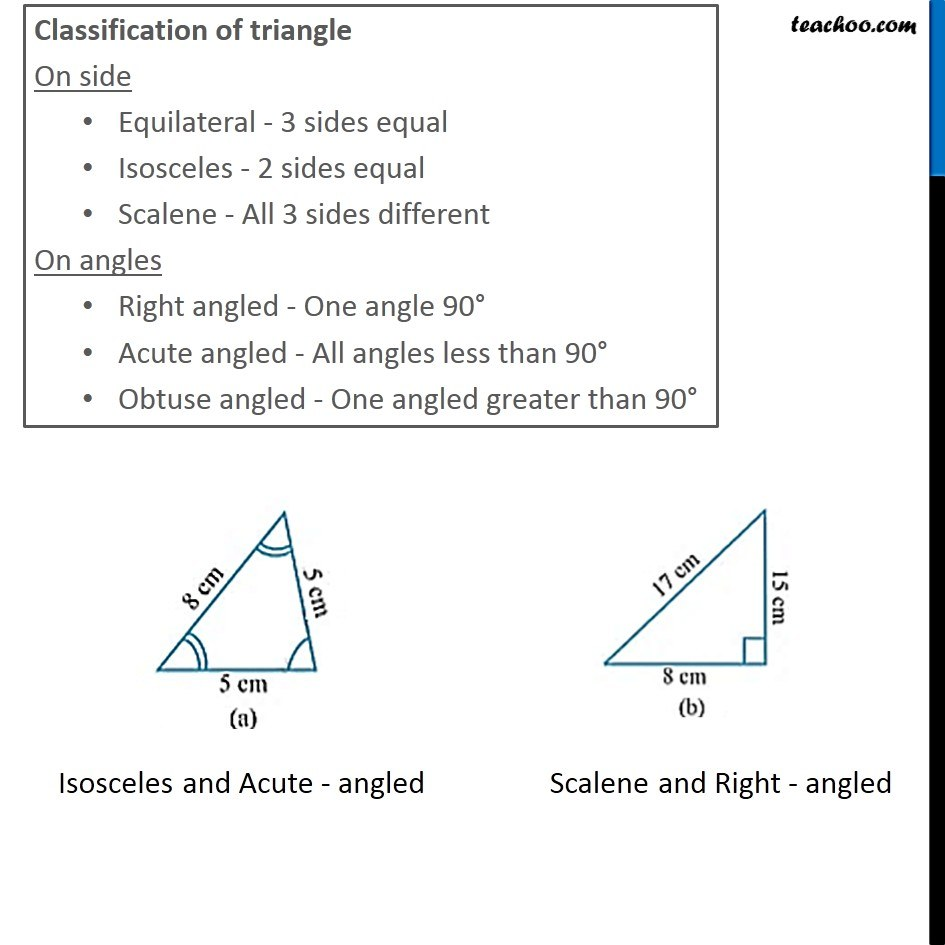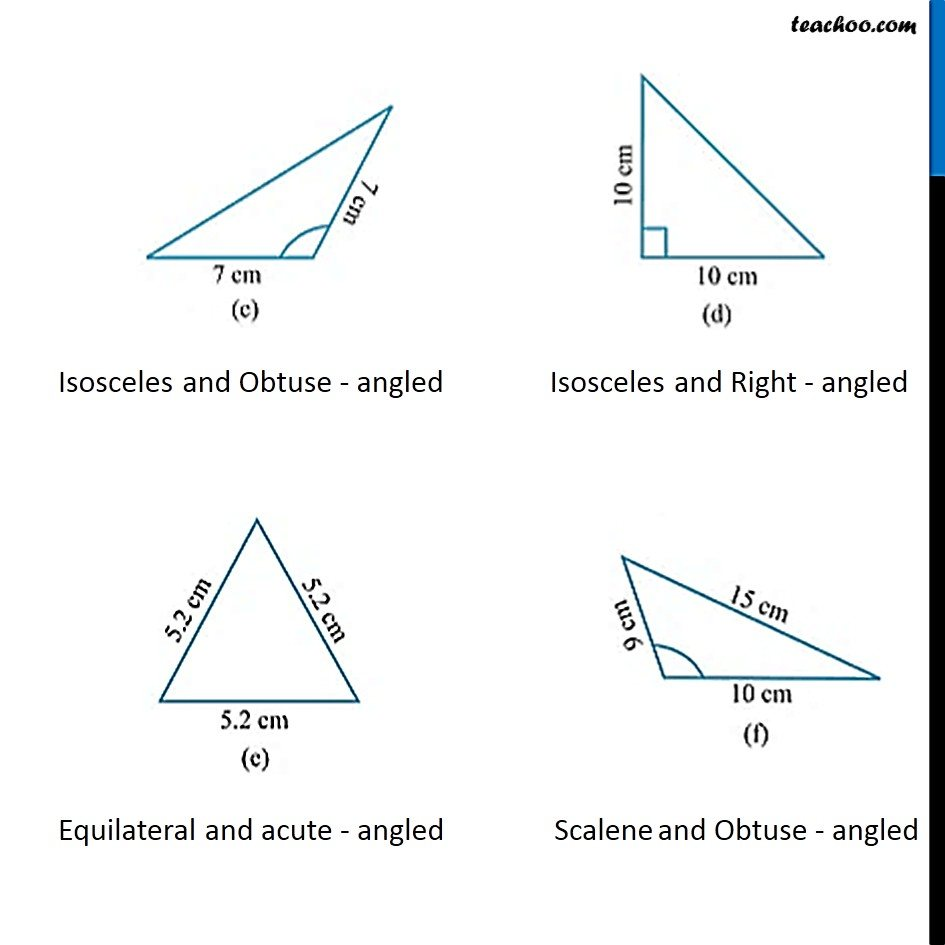1. Chapter 5 Class 6 Understanding Elementary Shapes
2. Concept wise
3. Classifying triangles

Transcript

Ex 5.6, 3 Name each of the following triangles in two different ways: (you may judge the nature of the angle by observation) Classification of triangle On side Equilateral - 3 sides equal Isosceles - 2 sides equal Scalene - All 3 sides different On angles Right angled - One angle 90° Acute angled - All angles less than 90° Obtuse angled - One angled greater than 90°

Classifying triangles